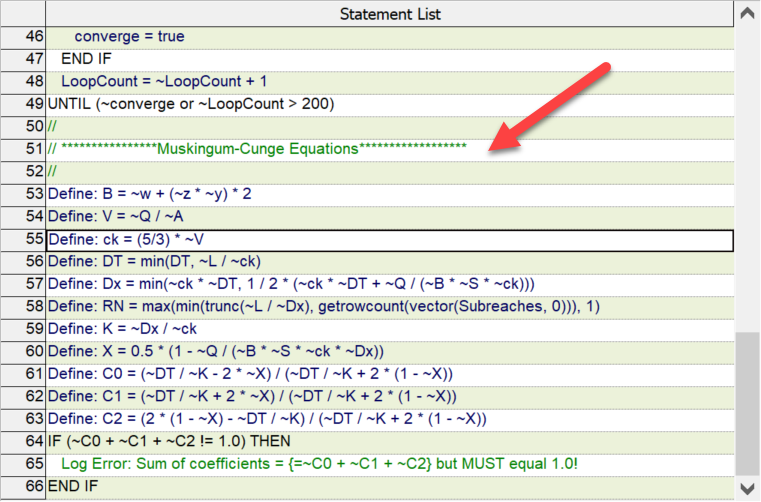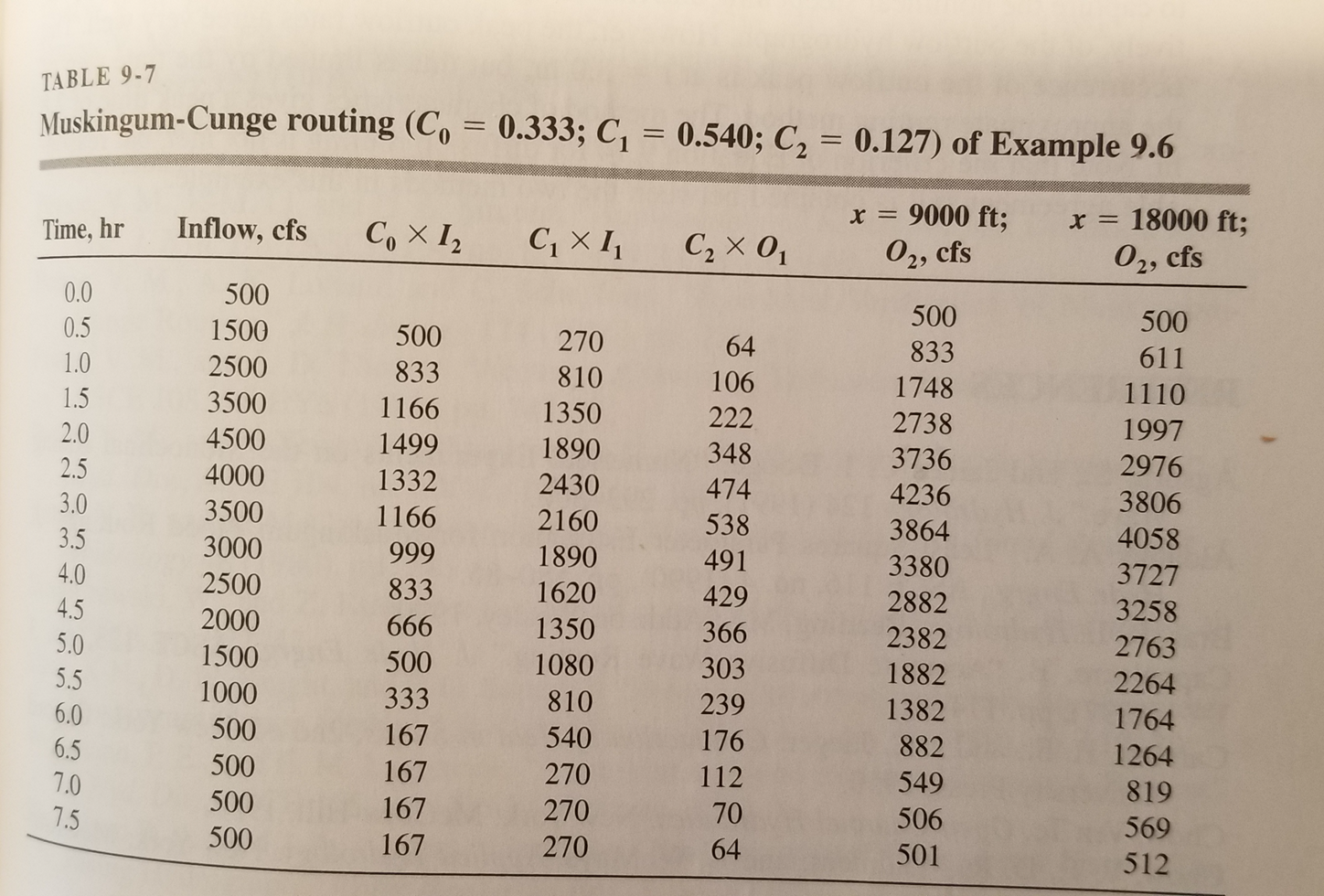•Jason

Thank you for posting in the GoldSim Community Forum. I will have a look at this model early next week and get back with you then. I hope this is timely enough for you! Thanks for your patience.

-Jason

•E216213

Hello Jason. Yes that is ok. Hope to hear from you soon!

•Jason

I started looking at the model and wanted to clarify a couple of things to make sure I understand the problem. First, just to make sure others reading this post understand: we are talking about this example model from our library: River Routing: Muskingum-Cunge Model – GoldSim Help Center

Next, I was wondering if you happened to have a chance to use the accompanying Excel model for comparison? The reason I ask is because the model was originally built with a comparison to the Excel model and I would be curious to know what result you see when using that version of the model to compare against GoldSim. You can obtain the Excel model using the link at the bottom of the before mentioned article. Is that something you might be able to compare before going to HEC-RAS?

•E216213

I have looked at the excel model you referred to and got similar results. The thing is all I need is the equality of the time of the peak outflow compared to Goldsim. Therefore I need to find how to shift the outflow graph.

Why do you think I get better results when I reduced the number of subreaches? How do I choose the number of subreaches?

•Jason

How do I choose the number of subreaches?

Go to the Model menu at the top of the application window, select Array Labels, then look for the "Subreaches" set in the list. Select the set and click the Edit Set... button. In here, you can change the number of subreaches.

After you get that fixed, please let me know if you are still experiencing issues. I'm not sure why you would have a shift in the results. Let's see what the change to the subreaches does and then we can proceed from there.

•E216213

Hi Jason,

I meant how to make the decision on the number of sub reaches - not how to put it on the array labels. When I reduced it from 20 to 5, I get better results.

•Jason

Ah I see. I built this model using the description outlined in the textbook "Open Channel Hydraulics", by Terry W. Sturm, 2001, McGraw-Hill. Unfortunately, I left this book at the office and won't be back in the office for a couple of weeks. But looking at the Script element where we define the variable "RN," which is the number of subreaches used in the routing functions. I see that we have the equation:

`max(min(trunc(~L / ~Dx), getrowcount(vector(Subreaches, 0))), 1)`

This appears that we calculate the subreaches as the minimum of L/Dx and the row count of the array label set. I would guess that as long as you make the array label set >= L / Dx, you should be okay. Please refer to the script element to see how Dx is defined.

Does this help?

-Jason

•E216213

hi Jason,

The thing is;  Dx is is calculated via script, and dependent on DT. that was the reason I asked at the beginning about how to choose DT. Therefore I can not decide beforehand building the model on the array set as >= L / Dx since I don't know the DX beforehand.

I tried some changes in script like; removing the min/max arguments and directly defining RN as L/Dx or removing the dependency of DX to DT, did not work. I can't seem to understand what makes the shift.

I also could take a look at the book you referenced and can't find the solution there as well. "https://vdocuments.mx/open-channel-hydraulics-sturm-569fb57a59d9c.html?page=371"

•Jason

I'll be in my office tomorrow and I can look in the textbook to give you a better answer.

-Jason

•Jason

I finally found my textbook. Are you still wondering how to get the value of Dx?

•E216213

Hi Jason! Yes very much :)

•Jason

I found a couple of updates were needed for the Script in the Muskingum Cunge model and updated it accordingly. The main change was renaming C1, C2, and C3 in the Script to C0, C1, and C2 to match the terminology in the textbook. I changed the name of c (line #55) to ck. Also, the example in the textbook assumes a wide channel so that ck = (5/3) * V (see line #55 of the Script).

According to the author of this example from the book,

If we consider the channel to be very wide as a first approximation, then ck = (5/3)*V.

Finally, I noticed that the order of C0 and C1 were swapped so I put them in the same order used in the textbook equation. This doesn't impact the result but clarifies the equations if you are comparing it to those used in the textbook.

Please use the new version going forward. It can be found here: https://support.goldsim.com/hc/en-us/articles/115016130888-River-Routing-Muskingum-Cunge-Model

If you look at the Script element in the GoldSim model, you will see the Muskingum-Cunge specific equations starting on line 51 of the Script.I'm using the same inputs as those used in the textbook:

`Qo = 2,500 cfs           # Approximate Reference Dischargew = 100 ft               # Channel Widthz = 2                    # Trapezoidal channel side slope (z : 1)n = 0.025                # Mannings Roughness CoeffL = 18,000 ft            # Overall Channel LengthSlope = 0.0005 ft/ft     # Channel SlopeDT = 0.5 hr              # Computation Time Increment`

In the latest version of this model, I set the value of DT = ~TimeStep_Length and found that the results still look good if I change the time step to something other than 30 minutes. Please have a look at the new model and see if you get the same result.

Using these inputs, we calculate the normal depth, y. This is used to calculate the flow area and then the channel flow velocity and finally the value of "ck" on line #55.

Using this, we can calculate the values of C0, C1, and C2, which are used to calculate the outflow hydrograph.

The Script assumes that the values of C0, C1, and C2 are constants, based on the "Reference Flow Rate" (Qo) mentioned above. Further, it uses a "Cn" parameter to calculate those, where Cn = DT / K. You can see how DT and K are calculated in the Script. Both of these are a function of DT as you stated but it should also be noted that on line #56 of the Script, DT could be reduced further as L / ck

The author of this example states,

If the value of Cn or X seems unsatisfactory, further slight adjustments of DT and Dx are possible since our equation for Dx is conservative.

I don't make any further adjustments to Cn in this model.

Using the expressions for C0, C1, and C2, we obtain:

`C0 = 0.333C1 = 0.539C2 = 0.128`

These values match those used in the textbook example. From this point, we use the C factors to transpose the hydrograph as done in the example:I verified that the GoldSim model results in exactly the same results as shown above.